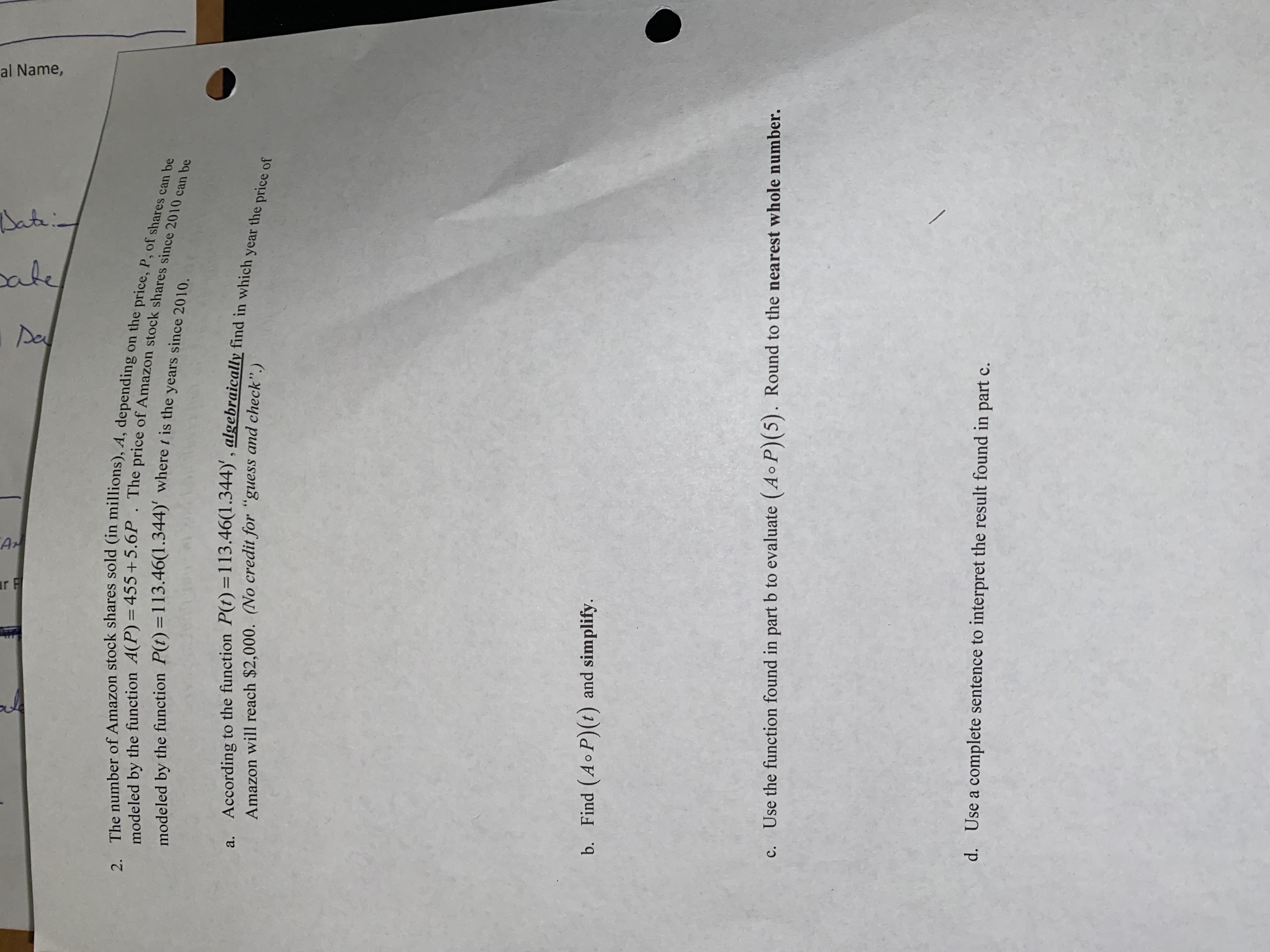# 2. The number of Amazon stock shares sold (in millions), A, depending on the price, P, of shares can bemodeled by the function A(P) = 455 +5.6P . The price of Amazon stock shares since 2010 can bemodeled by the function P(t)=113.46(1.344)' where t is the years since 2010.a. According to the function P(t)=113.46(1.344)', algebraically find in which year the price ofAmazon will reach \$2,000. (No credit for "guess and check".)Find (Ao P)(t) and simplify.b.Use the function found in part b to evaluate (Ao P)(5). Round to the nearest whole number.c.d.Use a complete sentence to interpret the result found inpart cal Name,SahaleDaArr F

Question
56 views

Composition of functions problemhelp_outlineImage Transcriptionclose2. The number of Amazon stock shares sold (in millions), A, depending on the price, P, of shares can be modeled by the function A(P) = 455 +5.6P . The price of Amazon stock shares since 2010 can be modeled by the function P(t)=113.46(1.344)' where t is the years since 2010. a. According to the function P(t)=113.46(1.344)', algebraically find in which year the price of Amazon will reach \$2,000. (No credit for "guess and check".) Find (Ao P)(t) and simplify. b. Use the function found in part b to evaluate (Ao P)(5). Round to the nearest whole number. c. d. Use a complete sentence to interpret the result found in part c al Name, Sah ale Da Ar r F fullscreen
check_circle

Step 1

Since the question contains multiple subparts and you have specifically asked for the solution for composition for functions problems i.e. subparts (b) and (c) only. So solving the subpart...

### Want to see the full answer?

See Solution

#### Want to see this answer and more?

Solutions are written by subject experts who are available 24/7. Questions are typically answered within 1 hour.*

See Solution
*Response times may vary by subject and question.
Tagged in

### Other